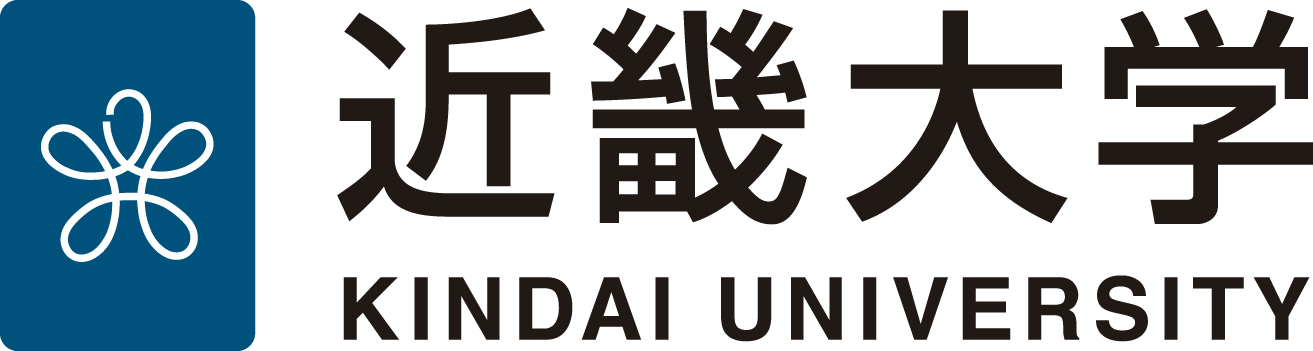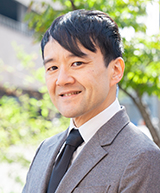#日本語*A space between the first name and last name, please enter

# SUZUKI Takao

## Profile

 ■Faculty Department of Science / Graduate School of Science and Engineering Research■Position Associate Professor ■Degree ■Commentator Guide https://www.kindai.ac.jp/meikan/524-suzuki-takao.html ■URL https://goo.gl/o4dfJ6 ■Mail
Last Updated :2020/04/05

## Education and Career

### Education

•   1992 04  - 1996 03 , Kobe University, Faculty of Science
•   1996 04  - 1998 03 , Kobe University, Graduate School of Science and Technology
•   2001 04  - 2004 03 , Kobe University, Graduate School of Science and Technology

•   2016 04 ,  - 現在, Associate Professor, Faculty of Science and Engineering, Kindai University
•   2012 04 ,  - 2016 03 , Lecturer, Faculty of Science and Engineering, Kindai University
•   2011 04 ,  - 2012 03 , Research Associate, Faculty of Liberal Arts and Sciences, Osaka Prefecture University

## Research Activities

### Research Areas

• Natural sciences, Mathematical analysis, Integrable system
• Natural sciences, Mathematical analysis
• Natural sciences, Basic analysis

### Research Interests

• Cluster algebra, Soliton equation, Painlev\'e equation, Integrable system, Discrete integrable system, Weyl group, Monodromy, Lie algebra, Garnier system, Root system, Hypergeometric function, Special function

### Published Papers

• A higher order Painlev\'e system in two variables and extensions of the Appell hypergeometric functions $F_1$, $F_2$ and $F_3$, Takao Suzuki, Funkcial. Ekvac., Funkcial. Ekvac., 61(1), 81 - 107, Apr. 2018 , Refereed
• A reformulation of the generalized $q$-Painlev\'{e} VI system with $W(A^{(1)}_{2n+1})$ symmetry, Takao Suzuki, J. Integrable Syst., J. Integrable Syst., 2(1), Feb. 2017 , Refereed
• A $q$-analogue of the Drinfeld-Sokolov hierarchy of type $A$ and $q$-Painlev\'{e} system, Takao Suzuki, AMS Contemp. Math., AMS Contemp. Math., 651, 25 - 38, Oct. 2015 , Refereed
• Higher order Painlev\'{e} system of type $D_{2n+2}^{(1)}$ and monodromy preserving deformation, Kenta Fuji, Keisuke Inoue, Keisuke Shinomiya, Takao Suzuki, J. Nonlinear Math. Phys., J. Nonlinear Math. Phys., 20, 57 - 69, Nov. 2013 , Refereed
• A class of higher order Painlev\'{e} systems arising from integrable hierarchies of type $A$, Takao Suzuki, AMS Contemp. Math., AMS Contemp. Math., 593, 125 - 141, Jul. 2013 , Refereed
• Higher order Painlev\'{e} systems of type $A$, Drinfeld-Sokolov hierarchies and Fuchsian systems, Takao Suzuki, Kenta Fuji, RIMS Kokyuroku Bessatsu, RIMS Kokyuroku Bessatsu, B30, 181 - 208, Apr. 2012 , Refereed
• A particular solution of a Painlev\'{e} system in terms of the hypergeometric function ${}_{n+1}F_n$, Takao Suzuki, SIGMA, SIGMA, 6, Oct. 2010 , Refereed
• Drinfeld-Sokolov hierarchies of type $A$ and fourth order Painlev\'{e} systems, Kenta Fuji, Takao Suzuki, Funkcial. Ekvac., Funkcial. Ekvac., 53(1), 143 - 167, Apr. 2010 , Refereed
• Coupled Painlev\'{e} VI system with $E_6^{(1)}$-symmetry, Kenta Fuji, Takao Suzuki, J. Phys. A: Math. Theor., J. Phys. A: Math. Theor., 42, Mar. 2009 , Refereed
• Higher order Painlev\'{e} system of type $D_{2n+2}^{(1)}$ arising from integrable hierarchy, Kenta Fuji, Takao Suzuki, Int. Math. Res. Not., Int. Math. Res. Not., 1, Jan. 2008 , Refereed
• The Lax pair for the sixth Painlev\'{e} equation arising from Drinfeld-Sokolov hierarchy, Takao Suzuki, Kenta Fuji, RIMS Kokyuroku Bessatsu, RIMS Kokyuroku Bessatsu, B2, 239 - 245, Mar. 2007 , Refereed
• The sixth Painlev\'{e} equation arising from $D_4^{(1)}$ hierarchy, Kenta Fuji, Takao Suzuki, J. Phys. A: Math. Gen., J. Phys. A: Math. Gen., 39, 12073 - 12082, Sep. 2006 , Refereed
• Classical solutions of the degenerate Garnier system and their coalescence structures, Takao Suzuki, J. Phys. A: Math. Gen., J. Phys. A: Math. Gen., 39, 12103 - 12113, Sep. 2006 , Refereed
• Affine Weyl group symmetry of the Garnier system, Takao Suzuki, Funkcial. Ekvac., Funkcial. Ekvac., 48(2), 203 - 230, Aug. 2005 , Refereed

### Books etc

• Representation Theory, Special Functions and Painlev\'e Equations — RIMS 2015, Hitoshi Konno, Hidetaka Sakai, Junichi Shiraishi, Takao Suzuki, Yasuhiko Yamada, 共編者(共編著者), Mathematical Society of Japan,   2018 06 , 9784864970501

### Conference Activities & Talks

• Affine Weyl groups and Painlev\'e type systems, Takao Suzuki, Microlocal Analysis and Asymptotic Analysis,   2019 11 21 , 招待有り
• Cluster algebra and $q$-Painlev\'{e} equations, Takao Suzuki, China-Japan Joint Workshop on Integrable Systems 2019,   2019 08 21
• A similarity reduction of $q$-Drinfeld–Sokolov hierarchy of type $A_{2n+1}^{(1)}$ and $q$-Garnier system, Takao Suzuki, Naoto Okubo, MSJ Annual Meeting,   2019 03 19
• Cluster algebra and generalized $q$-Painlev\'e VI systems of type $A$, Takao Suzuki, Conformal field theory, isomonodromy tau-functions and Painlev\'e equations, 2018,   2018 12 11 , 招待有り
• Cluster algebra and generalized $q$-Painlev\'e VI systems of type $A$, Takao Suzuki, Symmetries and Integrability of Difference Equations (SIDE) 13,   2018 11 13
• A similarity reduction of the Drinfeld-Sokolov hierarchy of type $A$ corresponding to the partition of natural number $(n+1,n+1,n+1)$, Takao Suzuki, MSJ Autumn Meeting,   2018 09 24
• A higher order generalization of the Painlev\'e VI equation with $W(A_{2n+1}^{(1)})$ symmetry, Takao Suzuki, Conformal field theory, isomonodromy tau-functions and Painlev\'e equations,   2017 12 01 , 招待有り
• A bilinear form of the generalized $q$-Painlev\'e VI system with $W(A^{(1)}_{2n+1})$ symmetry, Takao Suzuki, MSJ Autumn Meeting,   2017 09 11
• From Heine to $q$-Painlev\'e, Takao Suzuki, The 25th International Conference on Integrable Systems and Quantum symmetries,   2017 06 07
• A generalization of the $q$-Painlev\'e VI equation from a viewpoint of a basic hypergeometric solution, Takao Suzuki, Elliptic Hypergeometric Functions in Combinatorics, Integrable Systems and Physics,   2017 03 21
• From Heine to $q$-Painlev\'e, Takao Suzuki, Integrable Systems 2016,   2016 12 02
• A generalization of the $q$-Painlev\'e VI equation from a viewpoint of a basic hypergeometric solution, Takao Suzuki, Symmetries and Integrability of Difference Equations (SIDE) 12,   2016 07 08
• Fourth order $q$-Painlev\'e system containing $q$-hypergeometric function ${}_3\phi_2$, Takao Suzuki, MSJ Annual Meeting,   2016 03 18
• Higher order Painlev\'e systems, rigid systems and hypergeometric functions, Takao Suzuki, Analytic, Algebraic and Geometric Aspects of Differential Equations,   2015 09 14
• Drinfeld–Sokolov hierarchy of type $A_5^{(1)}$ and Simpson’s even four hypergeometric function, Takao Suzuki, MSJ Annual Meeting,   2015 03 24
• Higher order Painlev\'{e} systems, rigid systems and hypergeometric functions, Takao Suzuki, Recent developments in differential equations in the complex domain,   2014 11 17 , 招待有り
• Higher order Painlev\'{e} system, rigid system and hypergeometric function, Takao Suzuki, MSJ Annual Meeting,   2014 03 18 , 招待有り
• A $q$-analogue of the Drinfeld-Sokolov hierarchy of type $A$ and $q$-Painlev\'{e} system, Takao Suzuki, 2014 Joint Mathematics Meetings,   2014 01 18 , 招待有り
• A particular solution of the Schlesinger system $\mathcal{H}_{3,2}$ in terms of a rigid system, Takao Suzuki, MSJ Autumn Meeting,   2013 09 24
• Higher order Painlev\'{e} systems and hypergeometric functions, Takao Suzuki, The Eighth IMACS International Conference,   2013 03 25 , 招待有り
• 6-dimensional Painlev\'{e} equations and their particular solutions in terms of rigid equations, Takao Suzuki, MSJ Annual Meeting,   2013 03 22
• A class of higher order Painlev\'{e} systems arising from integrable hierarchies of type $A$, Takao Suzuki, Symmetry Plus Integrability 2010,   2010 06 14
• The Drinfeld-Sokolov hierarchies of type $A$: $q$-analogue and application to Painlev\'{e} systems, Takao Suzuki, Discrete Systems and Special Functions,   2009 06
• Higher order Painlev\'{e} system of type $D_{2n+2}^{(1)}$ arising from integrable hierarchy, Takao Suzuki, Kenta Fuji, International conference "From Painlev\'{e} to Okamoto",   2008 06
• Coupled Painlev\'{e} VI system arising from Drinfeld-Sokolov hierarchy, Takao Suzuki, An Isaac Newton Institute Workshop "Painlev\'{e} Equations and Monodromy Problems: Recent Developments",   2006 09
• The sixth Painlev\'{e} equation arising from $D_4^{(1)}$ hierarchy, Takao Suzuki, Symmetries and Integrability of Difference Equations (SIDE) VII,   2006 07 11
• The sixth Painlev\'{e} equation arising from $D_4^{(1)}$ hierarchy, Takao Suzuki, Kenta Fuji, Japanese-French Symposium "Algebraic, Analytic and Geometric Aspects of Complex Differential Equations and their Deformations. Painlev\'{e} Hierarchies",   2006 05

### Misc

• Generalized $q$-Painlev\'{e} VI systems of type $(A_{2n+1}+A_1+A_1)^{(1)}$ arising from cluster algebra, Naoto Okubo, Takao Suzuki,   2018 10 , https://arxiv.org/abs/1810.03252

### Research Grants & Projects

• JSPS, KAKENHI (Scientific Research (C)), Discretization of higher order Painlev\'e system and rigid system